themis contain extra steps for the recipes package for dealin gwith unbalanced data. The name themis is that of the ancient Greek god who is typically depicted with a balance.## Installation

You can install the released version of themis from CRAN with:

install.packages("themis")

Install the development version from GitHub with:

require("devtools")
install_github("tidymodels/themis")

## Example

Following is a example of using the SMOTE algorithm to deal with unbalanced data

library(recipes)
library(modeldata)
library(themis)

data(okc)

sort(table(okc$Class, useNA = "always")) #> #> <NA> stem other #> 0 9539 50316 ds_rec <- recipe(Class ~ age + height, data = okc) %>% step_meanimpute(all_predictors()) %>% step_smote(Class) %>% prep() sort(table(bake(ds_rec, new_data = NULL)$Class, useNA = "always"))
#>
#>  <NA>  stem other
#>     0 50316 50316

## Methods

Below is some unbalanced data. Used for examples latter.

example_data <- data.frame(class = letters[rep(1:5, 1:5 * 10)],
x = rnorm(150))

library(ggplot2)

example_data %>%
ggplot(aes(class)) +
geom_bar()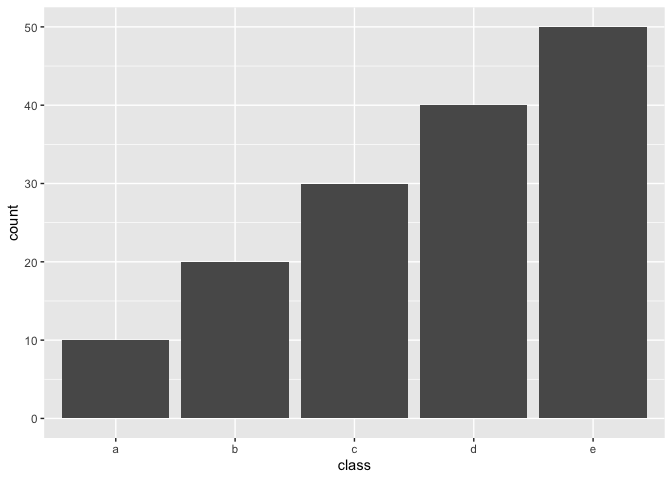### Upsample / Over-sampling

The following methods all share the tuning parameter over_ratio, which is the ratio of the majority-to-minority frequencies.

name function Multi-class
Random minority over-sampling with replacement step_upsample() :heavy_check_mark:
Synthetic Minority Over-sampling Technique step_smote() :heavy_check_mark:
Borderline SMOTE-1 step_bsmote(method = 1) :heavy_check_mark:
Borderline SMOTE-2 step_bsmote(method = 2) :heavy_check_mark:
Adaptive synthetic sampling approach for imbalanced learning step_adasyn() :heavy_check_mark:
Generation of synthetic data by Randomly Over Sampling Examples step_rose()

By setting over_ratio = 1 you bring the number of samples of all minority classes equal to 100% of the majority class.

recipe(~., example_data) %>%
step_upsample(class, over_ratio = 1) %>%
prep() %>%
bake(new_data = NULL) %>%
ggplot(aes(class)) +
geom_bar()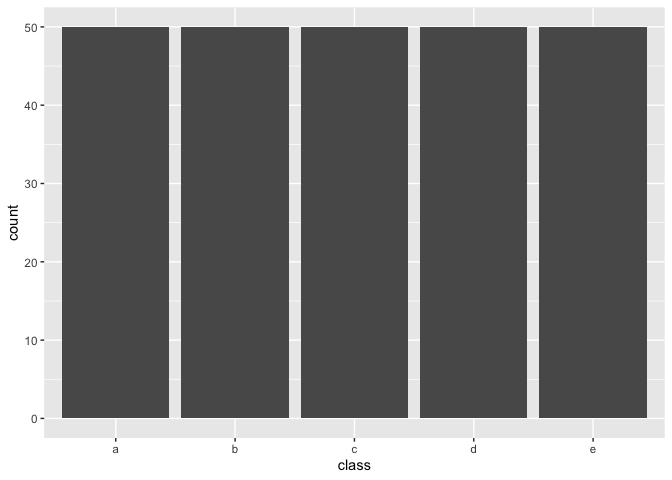and by setting over_ratio = 0.5 we upsample any minority class with less samples then 50% of the majority up to have 50% of the majority.

recipe(~., example_data) %>%
step_upsample(class, over_ratio = 0.5) %>%
prep() %>%
bake(new_data = NULL) %>%
ggplot(aes(class)) +
geom_bar()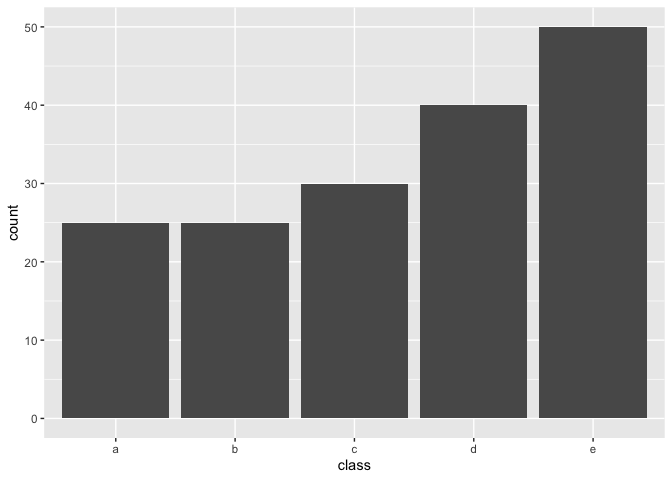### Downsample / Under-sampling

Most of the the following methods all share the tuning parameter under_ratio, which is the ratio of the minority-to-majority frequencies.

name function Multi-class under_ratio
Random majority under-sampling with replacement step_downsample() :heavy_check_mark: :heavy_check_mark:
NearMiss-1 step_nearmiss() :heavy_check_mark: :heavy_check_mark:
Extraction of majority-minority Tomek links step_tomek()

By setting under_ratio = 1 you bring the number of samples of all majority classes equal to 100% of the minority class.

recipe(~., example_data) %>%
step_downsample(class, under_ratio = 1) %>%
prep() %>%
bake(new_data = NULL) %>%
ggplot(aes(class)) +
geom_bar()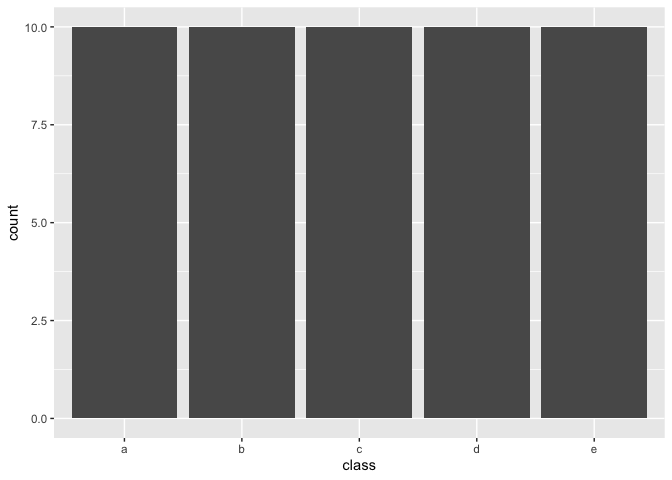and by setting under_ratio = 2 we downsample any majority class with more then 200% samples of the minority class down to have to 200% samples of the minority.

recipe(~., example_data) %>%
step_downsample(class, under_ratio = 2) %>%
prep() %>%
bake(new_data = NULL) %>%
ggplot(aes(class)) +
geom_bar()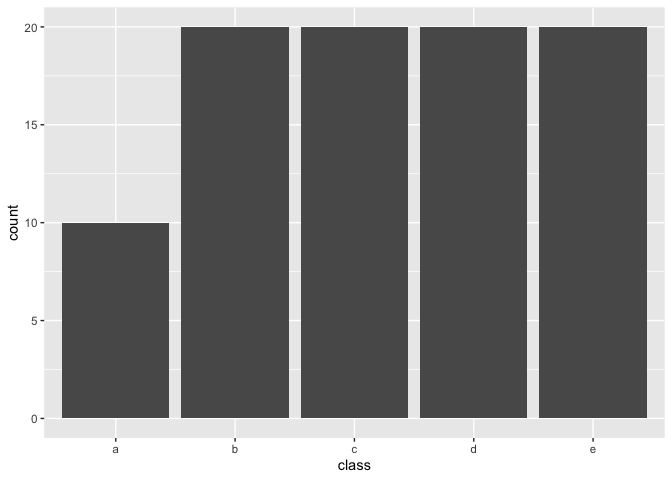## Contributing

This project is released with a Contributor Code of Conduct. By contributing to this project, you agree to abide by its terms.